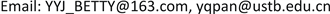1. 引言

2. 建模方法2.1. 理论阐述

2.2. 教育投资增长期权模型

Parameter definition of education investment growth optio

1) 工资W

d W t = α W t d t + σ W t d B t (1)

2) 未来要接受教育的时间T

3) 执行价格W0

4) 预期增长率e

5) 教育投资增长期权价值F(W)

1) 预期增长率e为常数，不随时间变化

2) 工资的价格变化遵循几何布朗运动

3) 人力资本的增长在受教育期间不能带来工资收入

4) 劳动力市场不存在无风险套利的行为

5) 市场上没有任何交易费用

6) 工资价格可以无限细分

F = W N ( d 1 ) − W 0 e − e t N ( d 2 ) (2)

d 1 = L n ( W / W 0 ) + ( e + σ 2 / 2 ) × T σ T − t (3)

d 2 = d 1 − σ T (4)

2.3. 市场潜力估计模型

(5)

ln y = ln a − e ( b − c t ) = ln a − e b e − c t (6)

S 1 = ∑ t = 1 r l n y t = r l n a − e b ∑ t = 1 r e − c t = r a − b e − c ( 1 − e − r c ) 1 − e − c (7)

S 2 = ∑ t = r + 1 2 r l n y t = r l n a − e b e − ( r + 1 ) c ( 1 − e − r c ) 1 − e − c (8)

S 3 = ∑ t = 2 r + 1 3 r l n y t = r l n a − e b e − ( 2 r + 1 ) c ( 1 − e − r c ) 1 − e − c (9)

a = S 1 r + b e − c ( 1 − e − r c ) r ( 1 − e − c ) , b = l n ( l n S 2 − l n S 1 ( 1 − e − r c ) 2 ( 1 − e − c ) ) , c = 1 r l n S 2 − S 1 S 3 − S 2 (10)

E = F × N (11)

3. 实证研究

3.1. 教育增长期权定价

Statistics on salary growth featur

Parameters of education growth optio

Education growth option pric

3.2. 行业增长潜力分析

l n y t = l n 5.2743 − e − 2.8358 e 0.0315 t (12)

l n y t = l n 4.4255 − e − 2.8701 e − 0.0524 t (13)

3.3. 教育现金流估计

Course opening schedul

4. 结论